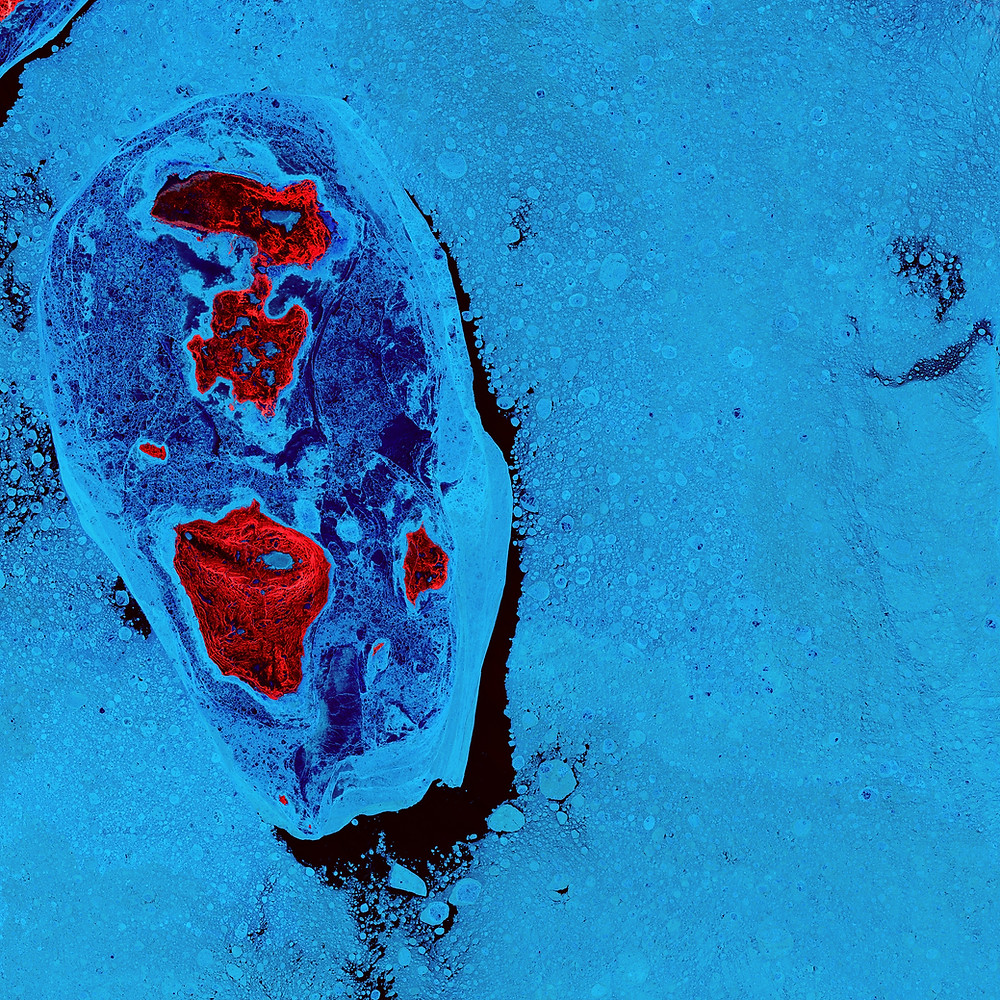Search

# Adaptive threshold, Threshold, Canny Image Filter In Machine learning OpenCV | CodersartsIt is used to solve the problem of a simple threshold were the problem for the different region which has different lighting values. Basically, adaptive thresholding is used to calculate the threshold value of smaller regions, it will be different threshold values for different regions.

This method used in machine learning OpenCV to clarify the visual effects of images.

In OpenCV, the adaptive threshold method is used is:

Syntax:

`adaptiveThreshold(src, dst, maxValue, adaptiveMethod, thresholdType, blockSize, C)`

Parameters:

There are different types of parameters which is used in “adaptiveThreshold” methods are as:

src: Input image array, source 8-bit single-channel image

dst: destination image of the same size and the same type as src.

maxValue: Maximum value that can be assigned to a pixel.

adaptiveMethod: A variable of integer the type representing the adaptive method to be used

It used two types of values:

threshodType: A variable of integer type representing the type of threshold to be used

blockSize: A variable of the integer type representing size of the pixelneighborhood used to calculate the threshold value.

C: A variable of double type representing the constant used in the both methods (subtracted from the mean or weighted mean).

### Threshold Image Filter

This method is simple and straightforward, If pixel value is greater than a threshold value, it is assigned one value (maybe white), else it is assigned another value (may be black).

For better understating, lets we suppose the threshold value is 125(out of 255), then value is 125 or under 125 can be converted to black or 0.

And if the value is above 125 can be converted to white or 1.

Syntax:

`threshold (src, dst, thresh, maxval, type)`

Parameters:

src: Input image array, source 8-bit single-channel image

dst: destination image of the same size and the same type as src.

thresh: threshold value

maxval: Maximum value that can be assigned to a pixel.

type: thresholding type

There are different types of threshold types:

• THRESH_BINARY

• THRESH_BINARY_INV

• THRESH_TRUNC

• THRESH_TOZERO

• THRESH_OTSU

• THRESH_TRIANGLE

### Canny Image Filter

Canny Edge Detection is a popular edge detection algorithm. It was developed by John F. Canny in 1986.

Canny Edge Detection is used to detect the edges in an image. It accepts a grayscale image as input and it uses a multi-stage algorithm.

Canny edge detection process:

It uses four steps to detect the edge:

• Noise Reduction - 5x5 Gaussian filter

• No maximum suppression - upper threshold

• Thresholding with hysteresis - upper/lower threshold

Syntax:

`Canny(image, edges, threshold1, threshold2)`

Parameters:

image: A object representing the source (input image) for this operation.

edges: A object representing the destination (edges) for this operation.

threshold1: A variable of the type double representing the first threshold for the hysteresis procedure.

threshold2: A variable of the type double representing the second threshold for the hysteresis procedure.

Get instant help in any types of programming and project assignment at "coderstarts" with the affordable prices, contact us, or send your requirement: